Processing ......FreeComputerBooks.com Links to Free Computer, Mathematics, Technical Books all over the World

Statistical Inference via Data Science: A ModernDive into R and the Tidyverse
Top Free Machine Learning Books 🌠 - 100% Free or Open Source!
• Title: Statistical Inference via Data Science: A ModernDive into R and the Tidyverse
• Author(s) Chester Ismay and Albert Y. Kim
• Publisher: Chapman and Hall/CRC; 1st edition (2019); eBook (Creative Commons Licensed, July 26, 2023)
• License(s): CC BY-NC-ND 3.0 US
• Hardcover/Paperback: 430 pages
• eBook: HTML
• Language: English
• ISBN-10/ASIN: 0367409879
• ISBN-13: 978-0367409876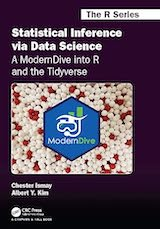Book Description

This book provides a pathway for learning about statistical inference using data science tools widely used in industry, academia, and government. It introduces the tidyverse suite of R packages, including the ggplot2 package for data visualization, and the dplyr package for data wrangling.

• Chester Ismay is a Data Science Evangelist for DataRobot and is based in Portland, Oregon, USA.
Reviews, Rating, and Recommendations: Related Book Categories: Read and Download Links:Similar Books:
•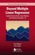Beyond Multiple Linear Regression: Linear and Multilevel Models

This book is designed for undergraduate students who have successfully completed a multiple linear regression course, helping them develop an expanded modeling toolkit that includes non-normal responses and correlated structure.

•Statistical Inference: Algorithms, Evidence, and Data Science

A masterful guide to how the inferential bases of classical statistics can provide a principled disciplinary frame for the data science of the twenty-first century. Every aspiring data scientist should carefully study this book, use it as a reference.

•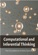Computational and Inferential: The Foundations of Data Science

Step by step, you'll learn how to leverage algorithmic thinking and the power of code, gain intuition about the power and limitations of current machine learning methods, and effectively apply them to real business problems.

•Statistical Inference for Everyone (Brian S Blais)

Approaching an introductory statistical inference textbook in a novel way, this book walks through a simple introduction to probability, and then applies those principles to all problems of inference.

•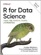R for Data Science: Visualize, Model, Transform, Tidy, Import

This book teaches you how to do data science with R: You'll learn how to get your data into R, get it into the most useful structure, transform it, visualize it and model it, how data science can help you work with the uncertainty and capture the opportunities.

•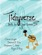Tidyverse Skills for Data Science (Carrie Wright, et al)

This book is intended for data scientists with some familiarity with the R programming language who are seeking to do data science using the Tidyverse family of packages, covers the entire life cycle of a data science project and presents specific tidy tools for each stage.

•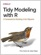Tidy Modeling with R: A Framework for Modeling in the Tidyverse

This book shows data analysts, business analysts, and data scientists how the tidymodels framework offers a consistent, flexible approach for your work. It demonstrate ways to create models by focusing on an R dialect called the Tidyverse.

•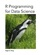R Programming for Data Science (Roger D. Peng)

This book is about the fundamentals of R programming. Get started with the basics of the language, learn how to manipulate datasets, how to write functions, and how to debug and optimize code. You will have a solid foundation on data science toolbox.

•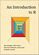An Introduction to R (Alex Douglas, et al.)

The main aim of this book is to help you climb the initial learning curve and provide you with the basic skills and experience (and confidence!) to enable you to further your experience in using R.

•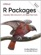R Packages: Organize, Test, Document, and Share Your Code

Turn your R code into packages that others can easily download and use. This practical book shows you how to bundle reusable R functions, sample data, and documentation together by applying author's package development philosophy.

•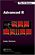Advanced R Programming (Hadley Wickham)

This book presents useful tools and techniques for attacking many types of R programming problems. It not only helps current R users become R programmers but also shows existing programmers what's special about R.

•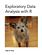Exploratory Data Analysis with R (Roger D. Peng)

This book covers the essential exploratory techniques for summarizing data with R. These techniques are typically applied before formal modeling commences and can help inform the development of more complex statistical models.

•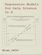Regression Models for Data Science in R (Brian Caffo)

The book gives a rigorous treatment of the elementary concepts of regression models from a practical perspective. The ideal reader for this book will be quantitatively literate and has a basic understanding of statistical concepts and R programming.

•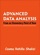Advanced Data Analysis using R (Cosma R. Shalizi)

This is a textbook on data analysis methods, intended for advance undergraduate students who have already taken classes in probability, mathematical statistics, and linear regression. All examples implemented using R.

•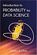Introduction to Probability for Data Science (Stanley Chan)

This book is an introductory textbook in undergraduate probability in the context of data science to emphasize the inseparability between data (computing) and probability (theory) in our time, with examples in both MATLAB and Python.

•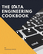Data Engineering Cookbook: The Plumbing of Data Science

This is a practical and comprehensive guide. You will learn the basics of data engineering. Then you will learn the technologies and frameworks required to build data pipelines to work with large datasets.

•Computational and Inferential: The Foundations of Data Science

Step by step, you'll learn how to leverage algorithmic thinking and the power of code, gain intuition about the power and limitations of current machine learning methods, and effectively apply them to real business problems.

•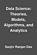Data Science: Theories, Models, Algorithms, and Analytics

It provides a bucket full of information regarding Data Science, covers a wide variety of sections by giving access to theories, data science algorithms, tools and analytics. You'll explore the right approach to best practices to guide you along the way.

•The Data Science Handbook: Advice and Insights

This book covers the essential exploratory techniques for summarizing data with R. These techniques are typically applied before formal modeling commences and can help inform the development of more complex statistical models.

•Python Data Science Handbook: Essential Tools (Jake VanderPlas)

Several resources exist for individual pieces of this data science stack, but only with the Python Data Science Handbook do you get them all - IPython, NumPy, Pandas, Matplotlib, Scikit-Learn, and other related tools.

Book Categories
 :All CategoriesTop Free BooksRecent BooksMiscellaneous BooksComputer EngineeringComputer LanguagesComputer ScienceData Science/DatabasesJava and Java EE (J2EE)Linux and UnixMathematicsMicrosoft and .NETMobile ComputingNetworking and CommunicationsSoftware EngineeringSpecial TopicsWeb Programming
Other Categories